# Accumulator Components

## Accumulator Component General Description

Anaccumulator is a pressure storage reservoir in which the fluid is held under pressure that is applied by an external source. The external source can be an engine, a spring, a raised weight, or a compressed gas. An accumulator enables a hydraulic system to cope with extremes of demand using a less powerful pump, to respond more quickly to a temporary demand, and to smooth out pulsations.

## Quick Guide for Creation in the GUI

There are two accumulator subtypes under “Compressible Gas Elements” section and five accumulator subtypes under “Incompressible Liquid Elements” section available in Flow Simulator.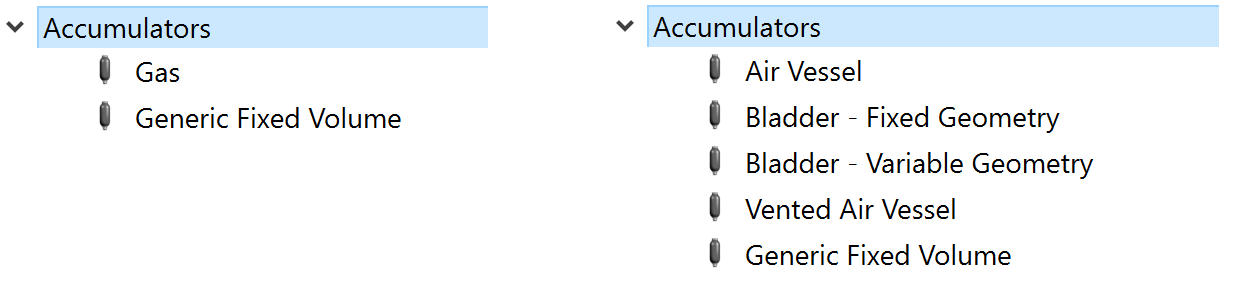## Accumulator Component Inputs

Table of the inputs for the Accumulator component.

 Element Specific Accumulator Component Input Variables Index UI Name (. flo label) Description 1 Type (CPTYPE) Type of component. See common component inputs table above for a full list. 2 Subtype (SUBTYPE) “Air Vessel” contains a liquid and a trapped gas. Elements carry the liquid in and out of the accumulator. The trapped gas is not in a bladder. “Bladder - Fixed Geometry” contains a liquid and a trapped gas in a bladder. Elements carry the liquid in and out of the accumulator. Only volume is needed to describe accumulator geometry “Bladder - Variable Geometry” contains a liquid and a trapped gas in a bladder. Elements carry the liquid in and out of the accumulator. Accumulator geometry can be described in detail using height and cross section area. “Gas” contains a gas and no liquid. Elements carry the gas in and out of the accumulator. “Vented Air Vessel” contains a liquid and a gas. Elements carry the liquid in and out of the accumulator. The gas can vent. The gas vent geometry and losses are described in the accumulator input. “Generic Fixed Volume” contains a liquid or a gas but not both (unless a 2-phase homogenous mixture is being used). Multiple elements carry the fluid in and out. 3 ASSOC_CHAM Chamber that is used to represent the fluid in the accumulator in the solver. 4 Geometry Type (GEOM_TYPE) Type of geometry inputs. Fixed Geometry: Constant cross section area or just volume Variable Geometry: Height vs Cross section area Variable Geometry: Height vs Volume 6 Accumulator Volume (TOTAL_VOLUME) The accumulator volume 8 Accumulator Cross-Section Area (CS_AREA) The accumulator cross section area 9 Accumulator Height (TOTAL_HEIGHT) Height of the accumulator 10 Base Level Elevation (BASE_LVL) Elevation at the bottom (base) of the accumulator. Used for pressure change due to gravity. 6 Liquid Type or Gas Type (FLUID_SPECIES) Identifies the type of fluid in the accumulator 7 Inlet Diameter (ARM1_DIAM) Opening diameter for the element attached to the accumulator 8 Gas Valve Inflow Diameter (VENT_IN_DIAM) Diameter of vent opening for air entering the accumulator. Only needed for subtype 5. 10 Gas Valve Outflow Diameter (VENT_EX_DIAM) Diameter of vent opening for air exiting the accumulator. Only needed for subtype 5. 11 Inflow Loss Coefficient (ARM1_INLET_K) Pressure loss coefficient for fluid entering the accumulator.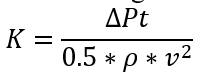12 Outflow Loss Coefficient (ARM1_EXIT_K) Pressure loss coefficient for fluid exiting the accumulator.13 Initial Mass Flow Rate or Volumetric Flow Rate (FLOW_RATE) A volumetric flow rate or mass flow rate used for the initial flow rate entering or leaving the accumulator. Behaviour depends on the accumulator subtype. 14 Polytropic Index (Inflow)(POLYTROPIC_IN) Polytropic Index for contracting gas (liquid flowing into the accumulator). 15 Polytropic Index (Outflow)(POLYTROPIC_EX) Polytropic Index for expanding gas (liquid flowing out of the accumulator). 16 Gas Pressure (GAS_PRESSURE) Initial gas pressure in the accumulator. 17 Gas Volume (GAS_VOLUME) Initial gas volume in the accumulator. 18 External Gas Pressure (EXT_PRESSURE) Ambient pressure for the vented gas in the Vented Air Vessel 19 Liquid Level (LIQ_LVL_OPT_1) Initial height of the liquid in the accumulator 20 Liquid Level When Air Valve Closed Liquid height at which the valve in the Vented Air Vessel will close. Liquid height below this level the valve is 21 Maximum Pressure (MAX_PRESSURE) This is not used in the solver. 22 Precharge Pressure (PRE_CHRG_PRESS) Pressure of gas in the bladder before any liquid is put in the accumulator. Its used to set the initial gas pressure if an initial gas volume is provided. 23 Gas Constant (GAS_CONSTANT) Gas property for the vented gas in the Vented Air Vessel 24 Specific Heat Ratio (SPECHEAT_RATIO) as property for the vented gas in the Vented Air Vessel. Also know as gamma. 25 Thermodynamic Process Type Gas expansion or contraction treatment. Polytropic process (enter index); Pres*Vol^index = constant Isentropic process (use fluid gamma); Pres*Vol^gamma = constant 26 HEIGHT Table of heights for the accumulator for the variable geometry option. Height values should be in ascending order and start at 0.0 and end at the maximum height of the accumulator. 27 CS_AREA Table of cross section areas of the accumulator for the variable geometry option. 28 VOLUME Table of volumes of the accumulator for the variable geometry option. Volume at height=0 should be 0. Other volumes should be total volume of the accumulator below the height.

## Accumulator Component Theory Manual

This section contains a governing equation of how mass, momentum & Energy conservation for all accumulator subtypes. Implicit Time Stepping solution is used to solve conservation equation as explained below.

 Nomenclature: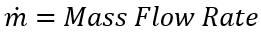V: volume A: cross section area gc: Gravitational Constant P: pressure R: gas constant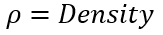T: Temperature V: volume t: time Cp: Specific Heat Subscripts: t: total liq: liquid s: static g: gas Superscripts: n: iteration

Generic Fixed Volume and Gas Chamber

Mass Conservation

Compressible Flow

Mass accumulated inside the control volume is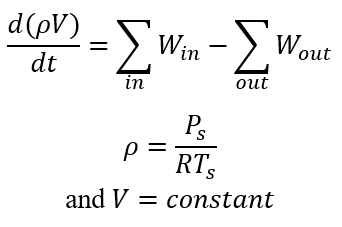Assuming first order backward time-stepping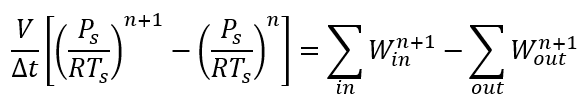Then, we define the residual equation similar to steady solver except for transient terms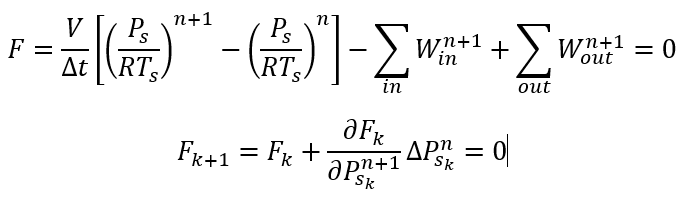We need to calculate: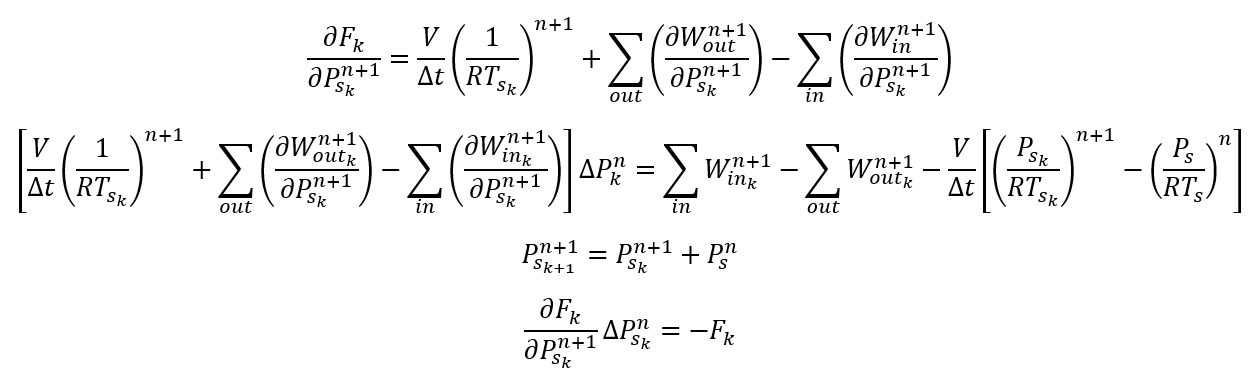For 2nd order central time stepping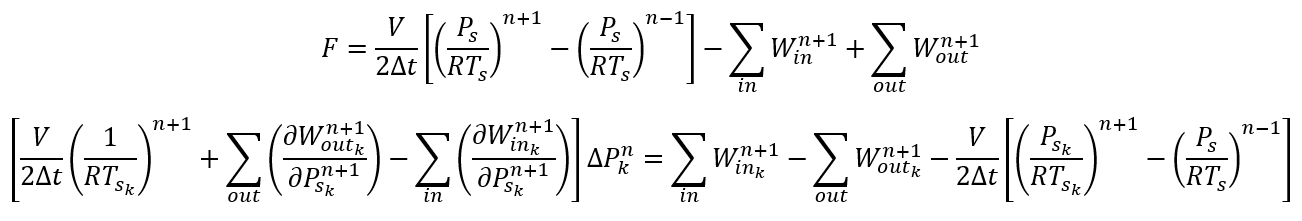Incompressible Flow

Mass accumulated inside the control volume is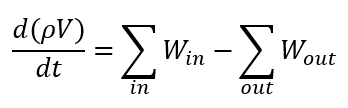Assuming first order backward time stepping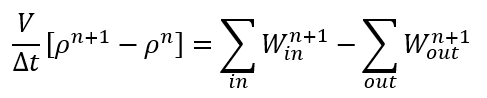Then, we define the residual equation similar to steady solver except for transient terms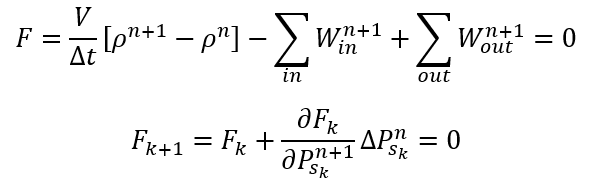Since density is a strong function of temperature and weak function of pressure, we assume temperature is independent of pressure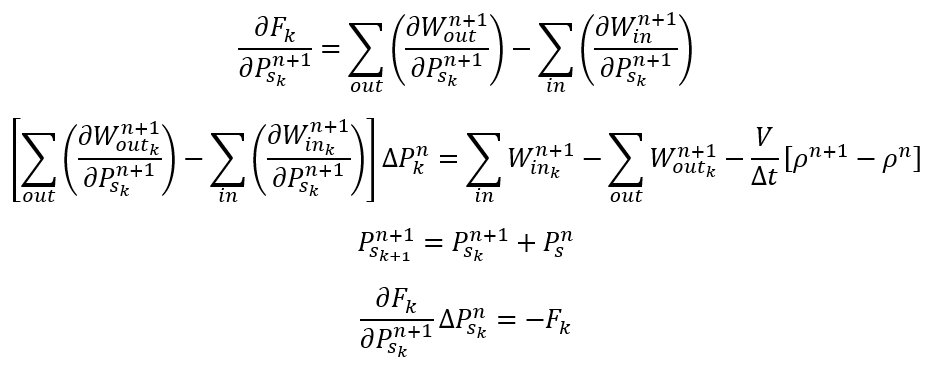For 2nd order central time stepping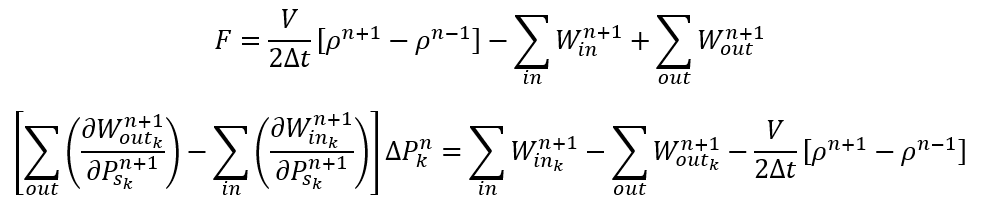Momentum conservation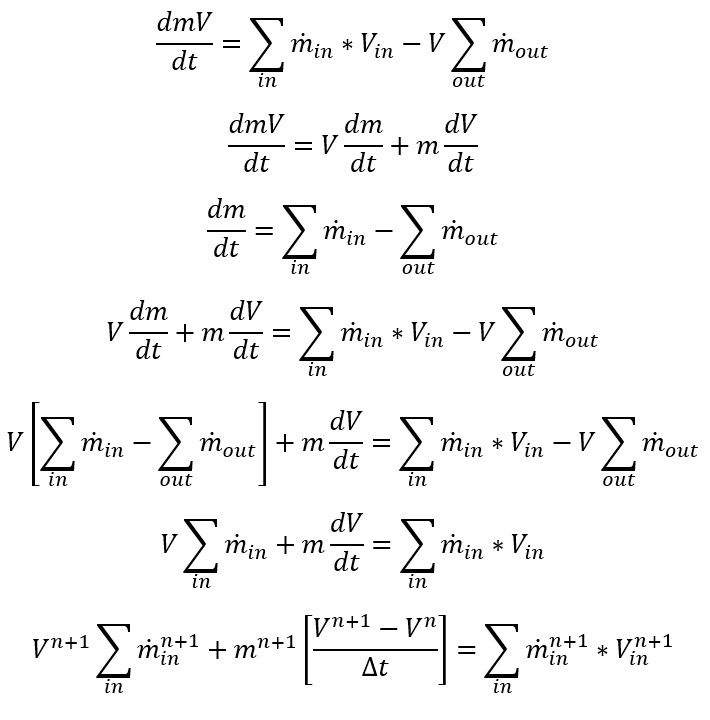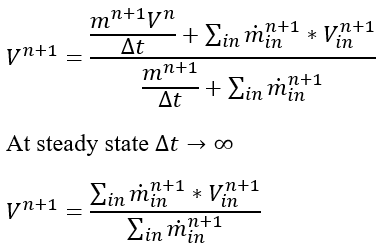Energy conservation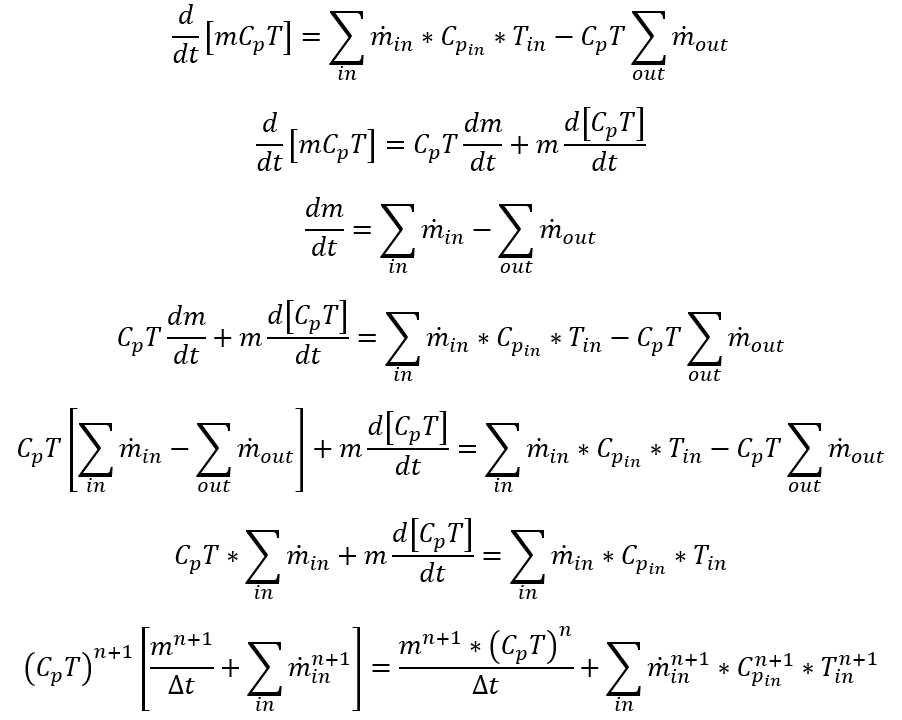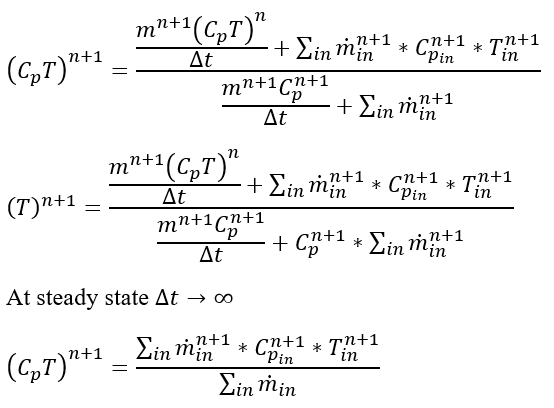Air Vessel, Vented Air Vessel, Bladder Fixed Geometry and Bladder Variable Geometry

The following derivations hold for all the following sub-types: Air Vessel, Vented Air Vessel, Bladder Fixed Geometry and Bladder Variable Geometry.

Mass Conservation

The continuity equation with the classical mass flux convention is given as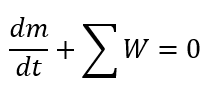Using a first order backward Euler time stepping, we get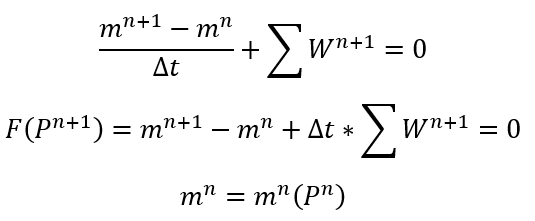Then the Jacobian matrix becomes: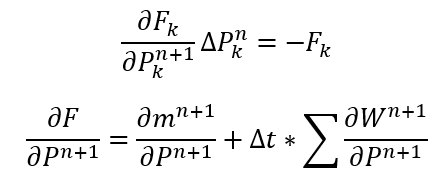Pressure at the exit of the accumulator will be given as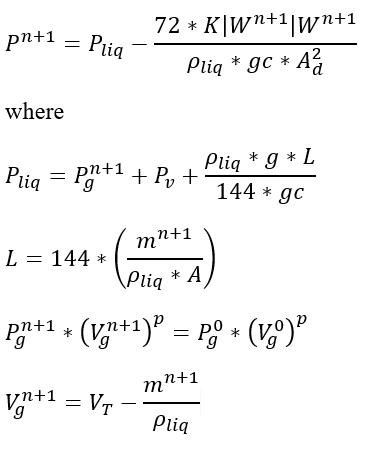For each time step and inner iteration, we should calculate mass. Since we have a single equation for 2 unknowns, we will assume the previous iteration’s flow rate while calculating the remaining mass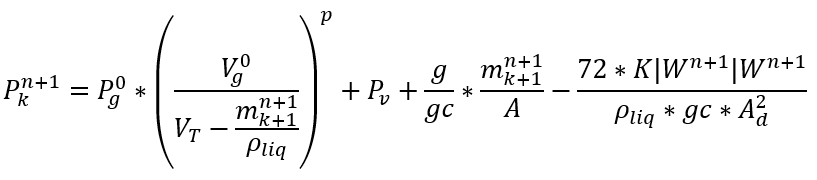Then, we have to solve for a non-linear equation for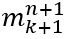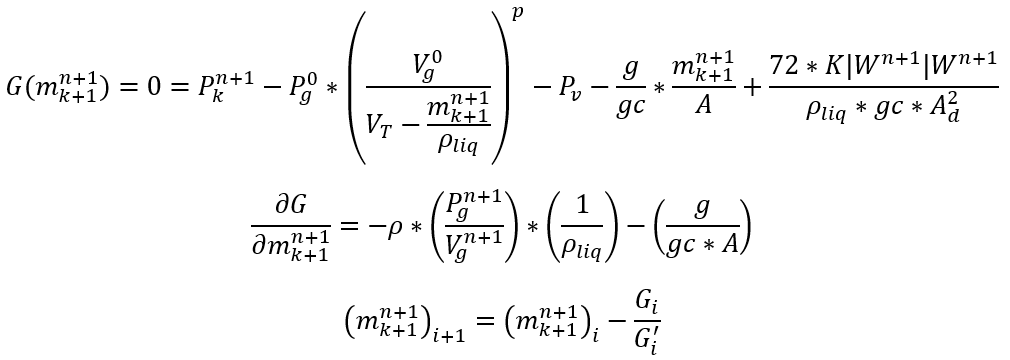G is the residual equation which needs to converge to a very small number.

Finding the mass inside the volume:

Now that we have defined the main residual equation, we will look at the calculation of remaining mass inside the volume. Since it will be mainly a function of h, or fluid height, the resulting equation will be in terms of h:

Case 1: Emptying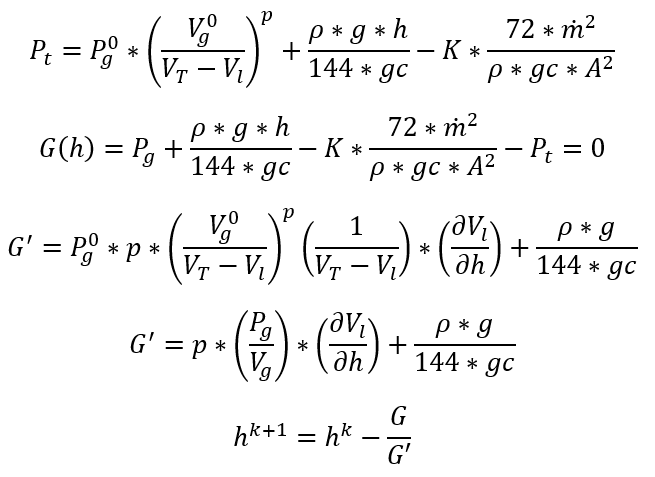Case 2: Filling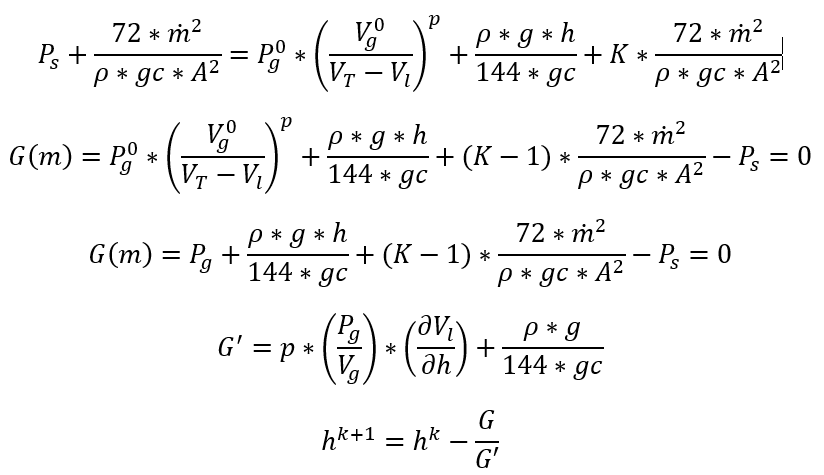Correcting the Continuity Equation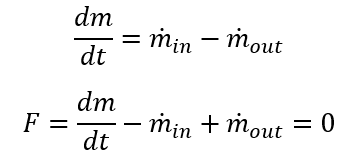First order implicit Finite Difference: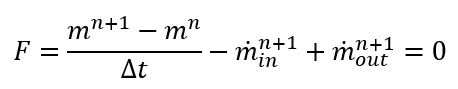Case 1: Emptying: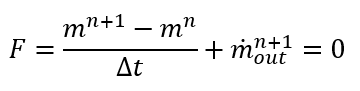Only contribution to Jacobian matrix will be on the off-diagonal term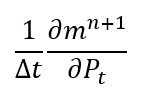Case 2: Filling: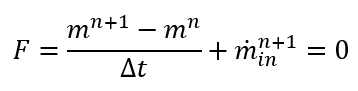Only contribution to Jacobian matrix will be on the off-diagonal term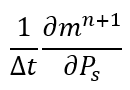Second order implicit Finite Difference: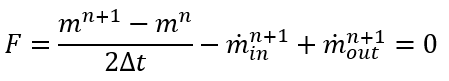Case 1: Emptying: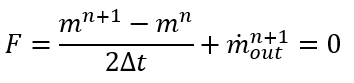Only contribution to Jacobian matrix will be on the off-diagonal term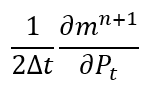Case 2: Filling: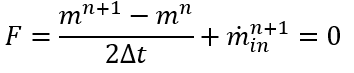Only contribution to Jacobian matrix will be on the off-diagonal term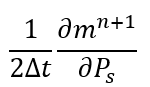Momentum and Energy Conservation

Case 1: Emptying Volume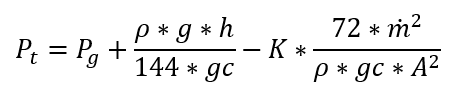In the above equation + if filling, and – if emptying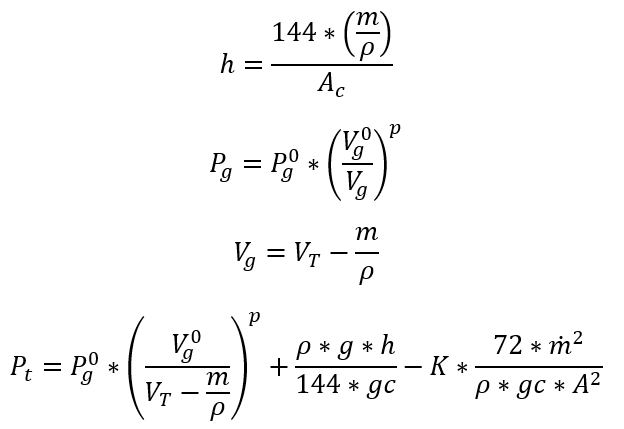Taking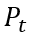derivative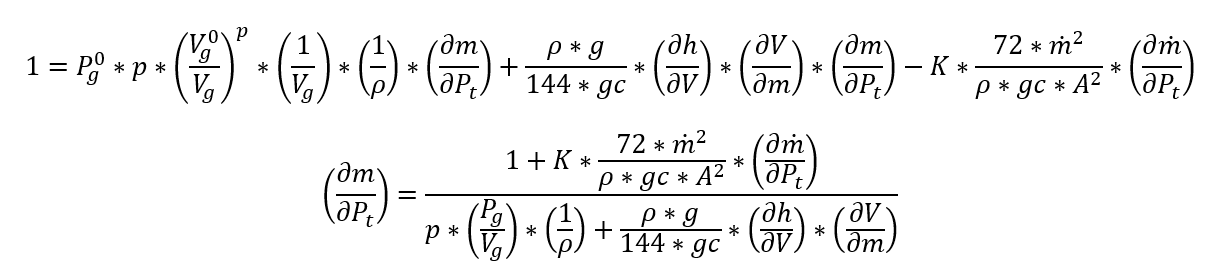Case 2: Filling Volume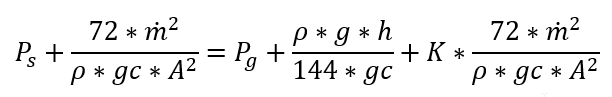In the above equation + if filling, and – if emptying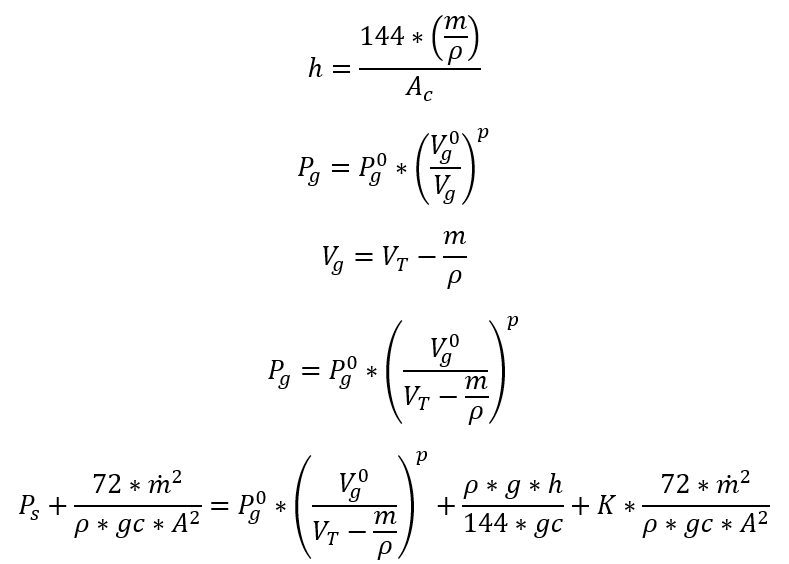Taking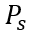derivative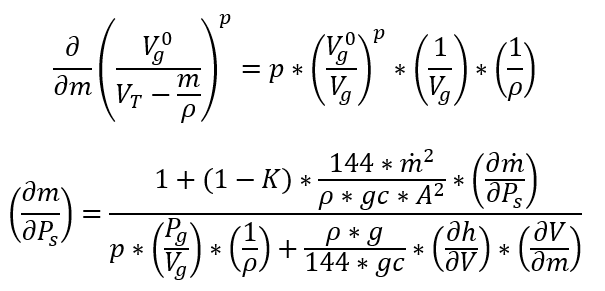Special Case: Ventilation Option for the Vented Air Vessel

If the ventilation is on, we need to calculate the mass of the trapped gas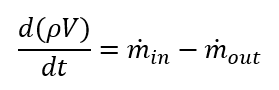If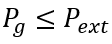, we will have in flow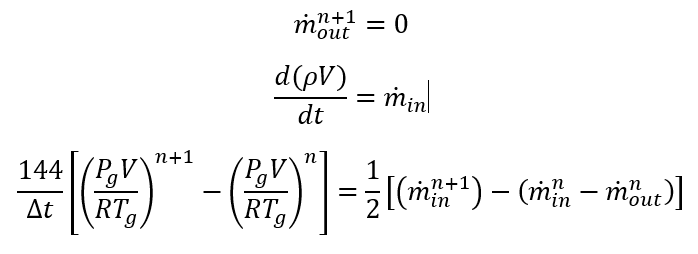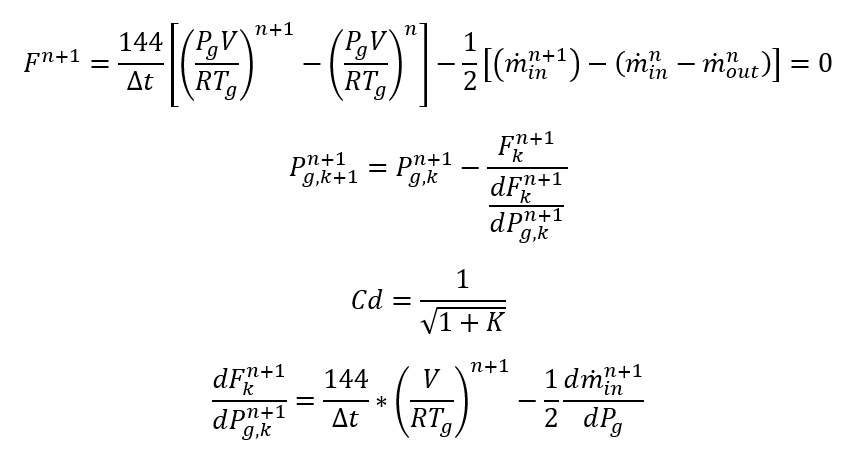If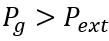, we will have out flow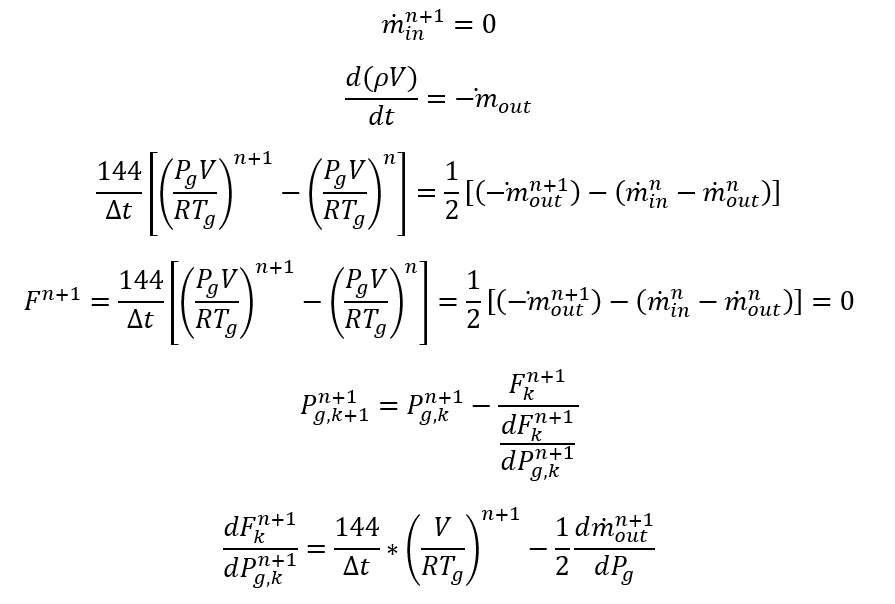## Accumulator Component Outputs

The following listing provides details about Accumulator Component output variables.

Name Description Units
ACCUMULATOR_COMPONENT Component ID Unitless
ASSOCIATED WITH CHAMBER

Chamber ID which Tank component is associated with

Unitless
SUBTYPE Subtype of the accumulator component Unitless
FLUID_TYPE Material type of the fluid Unitless
GAS & GENERIC FIXED VOLUME ACCUMULATOR
GAS MASS Mass of the gas lbm, kg
GAS MASS ACCUMULATION RATE Accumulation rate of the gas mass lbm/s, kg/s
GAS MASS FLOW RATE Gas mass flow rate lbm/s, kg/s
GAS PRESSURE Gas pressure psi, MPa
VENTED AIR VESSEL ACCUMULATOR
LIQUID MASS Mass of the liquid
LIQUID MASS ACCUMULATION RATE Accumulation rate of the liquid mass lbm/s, kg/s
LIQUID MASS FLOW RATE Liquid mass flow rate lbm/s, kg/s
GAS PRESSURE Gas pressure psi, MPa
GAS VOLUME Gas volume ft3, m3
LIQUID VOLUME Liquid volume ft3, m3
LIQUID LEVEL Liquid level in the accumulator in, m
BASE LEVEL Base level in the accumulator in, m